# How to render a scanline in VR space with Unity

## Summary

I explain how to render ‘scanline’, which looks like laser running on a surface, with Unity’s shader as shown below.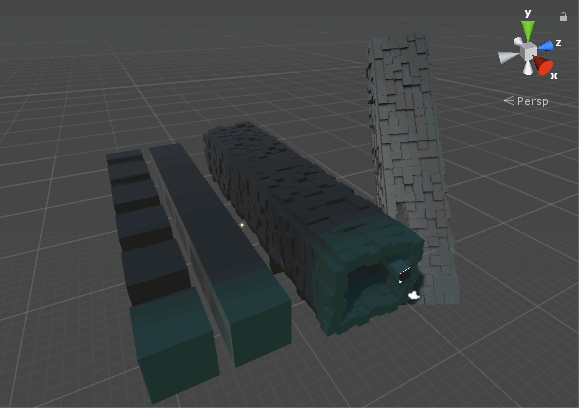Scanline in VR space

## View the sample asset by WebEditor

View the sample asset by WebEditor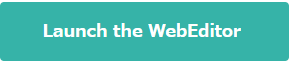※If you have your STYLY account, you can view the sample asset created in this article by WebEditor. If you don’t, create a new STYLY account and then press the button above.

You can create a new STYLY account >>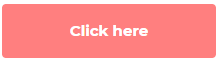## Samples

The sample available in GitHub: https://github.com/styly-dev/STYLY-Unity-Examples/tree/master/Assets/STYLY_Examples/WorldScanlineShader_Sample   The sample VR space: http://gallery.styly.cc/r-ngtm/c42952b6-e559-11e8-b34d-4783bb2170d0

## How to make scanline in VR space

I explain how to create a scanline in VR space.

### Use the x coordinate as colour

Unity’s shader takes the coordinate, where the pixel of the object on the screen is located, as a parameter. For example, if you used the x coordinate as the colour of the object,  it would look like the shown below.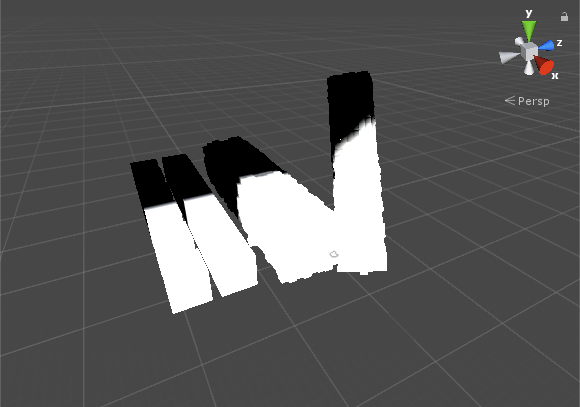Use the x coordinate as the colour of an object

The part where the X coordinate is smaller than 0.0 is coloured in black, where it is larger than 1.0 is coloured in white, and it’s coloured in grey in the case the X coordinate takes the value between 0.0 and 1.0.   The code for Unity’s ShaderLab is shown below.

```float x = IN.worldPos.x; // x coordinate
o.Emission = x; // Use x as colour```

### Create scanline

When the condition, 0.0 ≦ the X coordinate ≦ 0.1, is satisfied, colour the object white. Otherwise, colour it black.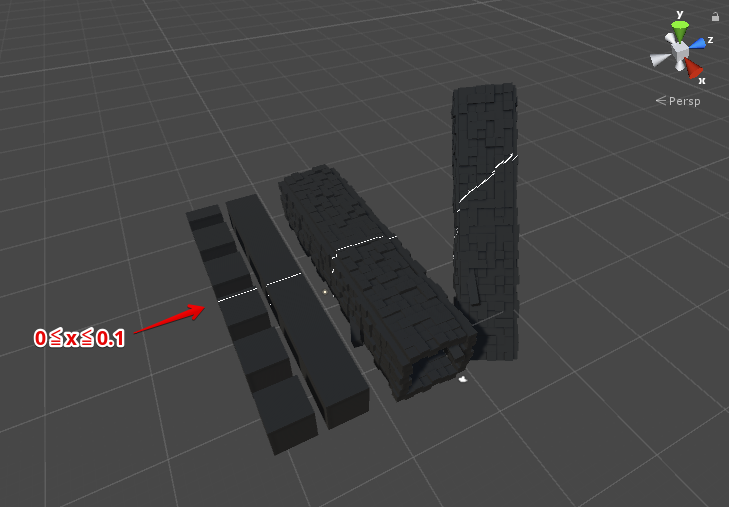The part with the condition, 0≦x≦0.1, is coloured white.

The code for Unity’s ShaderLab is shown below.

```float x = IN.worldPos.x; // x coordinate
o.Emission = step(0.0, x) * step(x, 0.1);  // Use x as colour```

Add a trace to the scanline.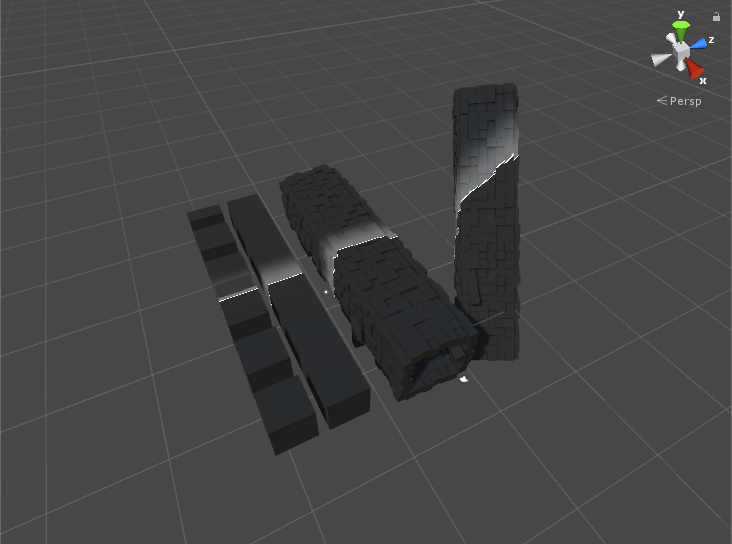Add a trace to the scanline.

The code for Unity’s ShaderLab is shown below.

```float x = IN.worldPos.x; // x coodinate
float scanline = step(0.0, x) * step(x, 0.1); // scanline
float trajectory = step(x, 0.0) * smoothstep(-4.0, 0.0, x); // trace of scanline
o.Emission = scanline + trajectory * 0.3; // output line and trace```

### Move Scanline

Create a series of scanlines and animate them.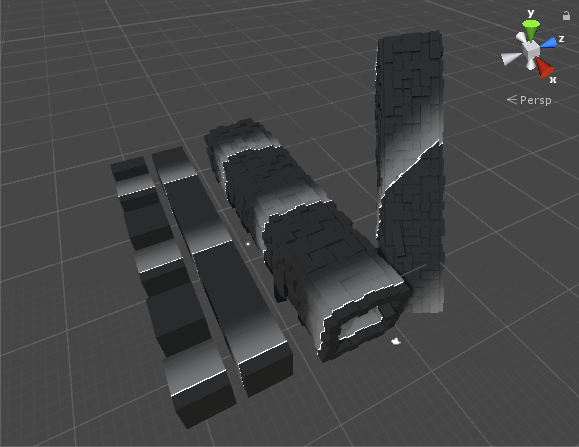Animate scanlines

The code for Unity’s ShaderLab is shown below.

```float x = IN.worldPos.x; // x coordinate
x -= _Time.z * 6.0; // subtract time from x coodinate
x = fmod(x, 12.0); // generate the float between 0.0 and 12.0
float scanline = step(-0.1, x) * step(x, 0.0); // scanline
float trajectory = step(x, 0.0) * smoothstep(-4.0, 0.0, x); // trace of scanline
o.Emission = scanline + trajectory * 0.3; // output as colour```

### Colour the scanlines ~ completion

Finally, colour the scanlines. Now we finished rendering the scanlines in VR space.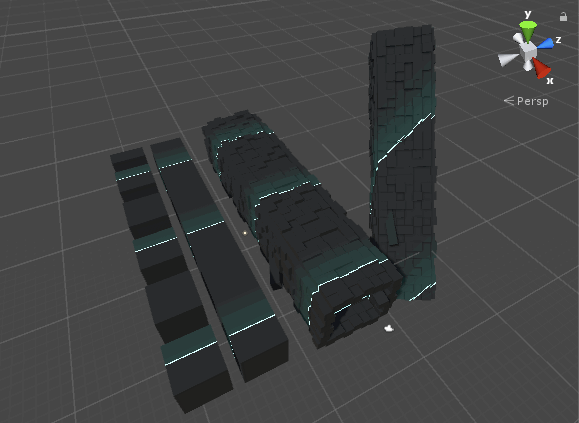Colour the scanlines

```float x = IN.worldPos.x; // x coordinate
x -= _Time.z * 6.0; // subtract time from x coodinate
x = fmod(x, 12.0); // generate the float between 0.0 and 12.0
float scanline = step(-0.1, x) * step(x, 0.0); // scanline
float trajectory = step(x, 0.0) * smoothstep(-4.0, 0.0, x); // trace of scanline
o.Emission = scanline * _LineColor + trajectory * _TrajectoryColor; // output as colour```

## Create Scanline with Unity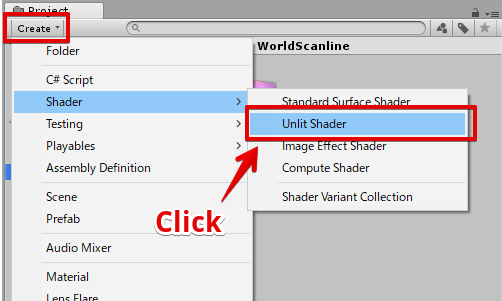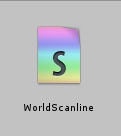Open the ‘WorldScanline’ shader, edit it as shown below.

```Shader "STYLY/Examples/WorldScanline" {
Properties{
_MainTex("Albedo (RGB)", 2D) = "black" {}
_LineColor("Scan Line Color", Color) = (1,1,1,1)
_TrajectoryColor("Scan Trajectory Color", Color) = (0.3, 0.3, 0.3, 1)
_LineSpeed("Scan Line Speed", Float) = 1.0
_LineSize("Scan Line Size", Float) = 0.02
_TrajectorySize("Scan Trajectory Size", Float) = 1.0
_IntervalSec("Scan Interval", Float) = 2.0

[Space]
[Space]
_Glossiness("Smoothness", Range(0,1)) = 0.5
_Metallic("Metallic", Range(0,1)) = 0.0
}
Tags { "RenderType" = "Opaque" }
LOD 200

CGPROGRAM
// Physically based Standard lighting model, and enable shadows on all light types

// Use shader model 3.0 target, to get nicer looking lighting
#pragma target 3.0

sampler2D _MainTex;

struct Input {
float2 uv_MainTex;
float3 worldPos;
};

// line parameters
fixed4 _LineColor; // color of line
half _LineSpeed; // speed of line movement
half _LineSize; // thickness of line

fixed4 _TrajectoryColor; // color of trajectory
half _TrajectorySize; // size of trajectory

half _IntervalSec; // interval(sec)

// other
half _Glossiness;
half _Metallic;

void surf(Input IN, inout SurfaceOutputStandard o) {

#define LINE_POS (_Time.w * _LineSpeed)
#define INTERVAL (_IntervalSec * _LineSpeed)
#define STEP_EDGE (_LineSize)
#define STEP_EDGE_1 (STEP_EDGE + _TrajectorySize)
#define STEP_EDGE_2 (STEP_EDGE)

float scanline = step(
fmod(abs(IN.worldPos.x - LINE_POS), INTERVAL),
STEP_EDGE
);
float trajectory = smoothstep(
STEP_EDGE_1, STEP_EDGE_2,
fmod(abs(IN.worldPos.x - LINE_POS), INTERVAL)
);

fixed4 baseColor = tex2D(_MainTex, IN.uv_MainTex);
o.Albedo = baseColor.rgb;
o.Emission = _LineColor * scanline + _TrajectoryColor * trajectory;
o.Metallic = _Metallic;
o.Smoothness = _Glossiness;
}
ENDCG
}
FallBack "Diffuse"
}
```

### Create a material

Right-click the shader file and select ‘Create > Material’ to create a material.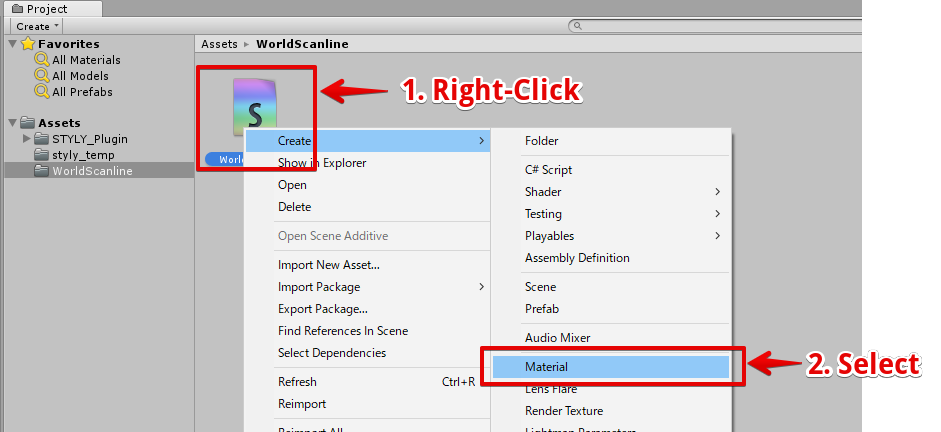Create a material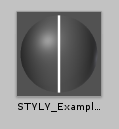The material created

### Assign the material to a Cube

In the Hierarchy window, select ‘Create > 3D Object  > Cube’ to create a Cube object.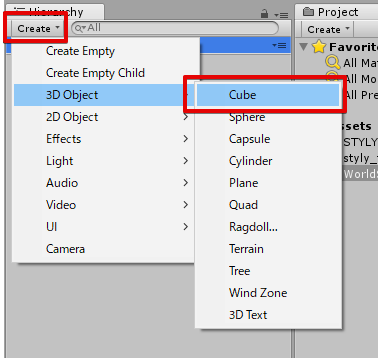Crate a Cube object.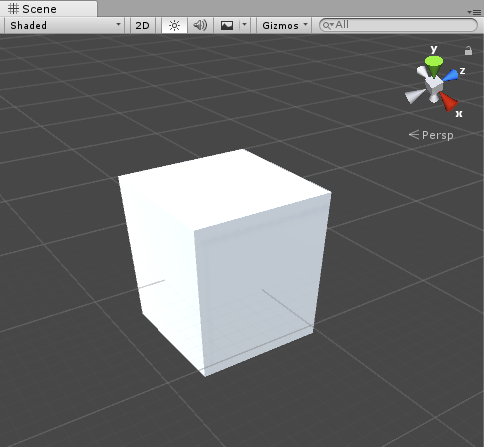The Cube object created.

Drag the material created earlier into the Cube to assign the material to it.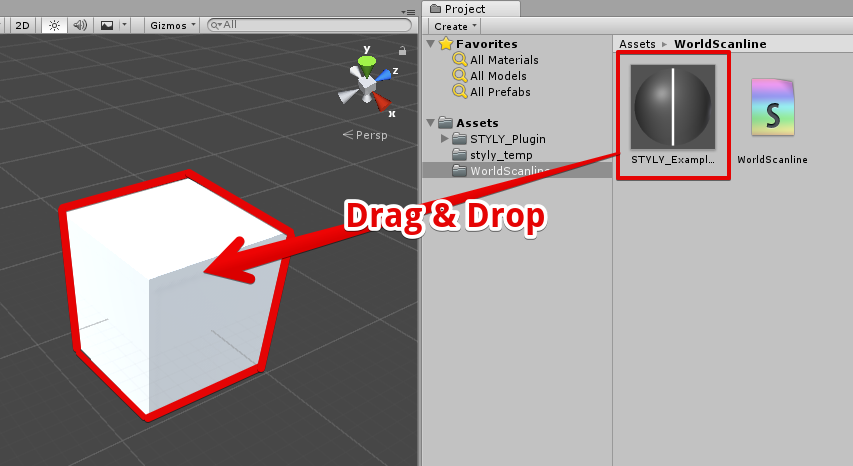Assign the material to the Cube object.

By playing the game with the scanline material applied, you can see the animated scanline on the Cube.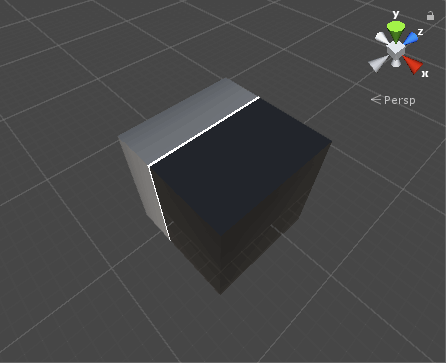The result – after applying the material

### Create a ‘Sci-Fi’ board

I explain how to create a board full of Sci-Fi atmosphere.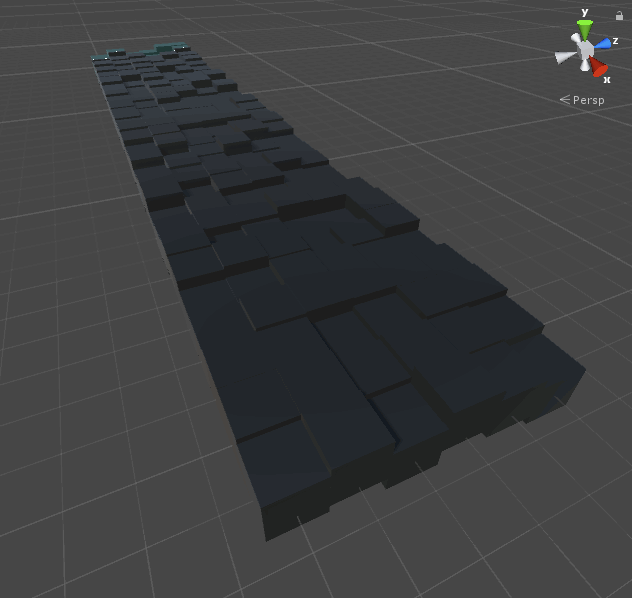Render a scanline on the cubes arranged like a board.

Firstly, arrange a lot of Cubes to form the board-like shape as shown below.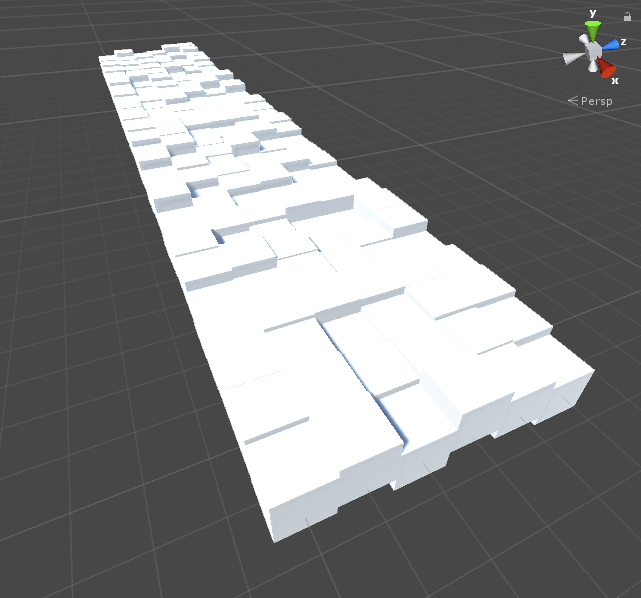Arrange Cubes

Set the parameters of the material as shown below and assign it to each cube.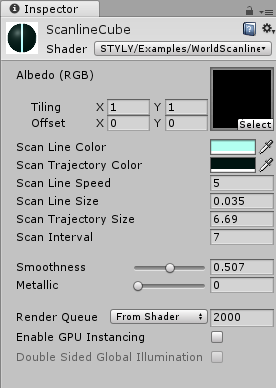The setting for the material.

Once the material has been applied, the appearance would be like the shown below.The result – after applying the scanline shader

## How to upload an asset from Unity to STYLY

Please refer to the article below to learn how to upload an asset from Unity to STYLY. https://styly.cc/ja/manual/unity-asset-uploader/